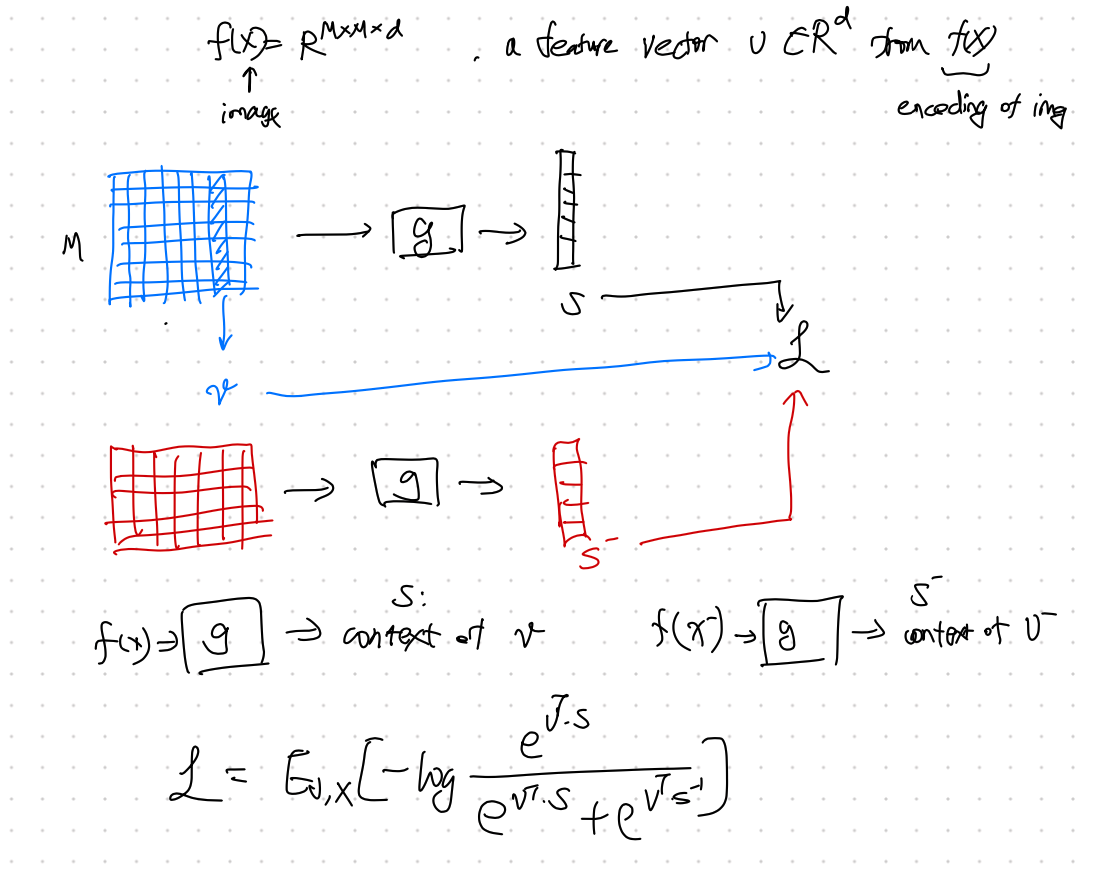In contrastive methods, we can manipulate the data to create data entries and infer the changes using a model. These methods are models that “predict relative position”1. Common tricks are

• shuffling image sections like jigsaw, and
• rotate the image.

We can also adjust the model to discriminate the similarities and differences. For example, to generate contrast, we can also use as the objective. Take two encoded space from the encoder, $g_1$ and $g_2$, we shall maximize the mutual information between the two representations if they are representing the same thing.

## Deep InfoMax

However, mutual information is hard to calculate. Models such as use instead1. For Deep InfoMax, the loss function is

$$\mathcal L = \mathbb E_{v, x} \left[ -\ln \frac{e^{v^T\cdot s}}{e^{v^T\cdot s} + e^{v^T\cdot s^-}} \right].$$Illustration of Deep InfoMax based on Liu2020.

Planted: by ;